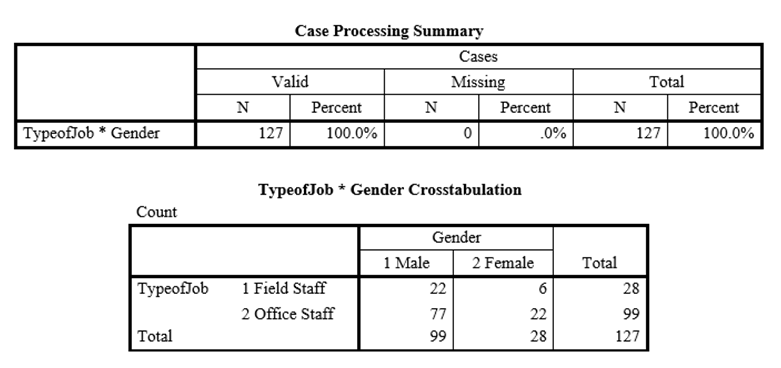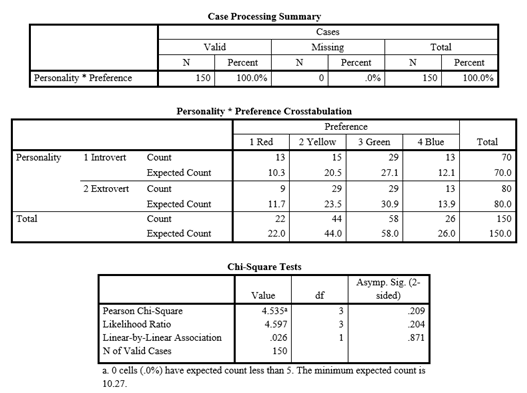# Crosstab Report and Chi-Square Test Using SPSS

## Crosstab Report using SPSS

Another way to describe categorical data is through the use of Crosstab report also located under Descriptive Statistics menu. Crosstab report mainly provide with number of occurrences (frequencies) in a tabular form.

• Crosstabulation helps produce summary results in the form of a table where data is grouped at an intersection point.
• The data in crosstab reports is summarized in the form of rows and columns.
• The Crosstabs procedure forms two-way and multiway tables (through Layers).
• Layer helps categorize the output. For instance, If you specify a row, a column, and a layer factor (control variable), the Crosstabs procedure forms one panel of associated statistics and measures for each value of the layer factor.
• For example, if gender is a layer factor for a table of married (yes, no) against life(is life exciting, routine, or dull), the results show a separate association between married and life for each gender.

### Steps involved in obtaining Crosstab Report

1. Choose Analyze Descriptive StatisticsCrosstabs.
2. Crosstabs dialog box is displayed, asking for data to be divided into row(s) and column(s), now it’s totally on you which variable you put in row or column, it is mostly based on how the data is more readable.
3. To find out percentages with each of the occurrences, you can select Cells button after inserting the variables in the row(s) and Column(s) list box. Once the dialog box is displayed, check rows, column or total from the percentages group box.
4. Press OK

A sample output generated from above steps is shown below.### Sample Interpretation of Crosstab Report

First table Case Processing Summary holds summary information. It shows that the sample had 127 respondents with no missing data. The second table is of main interest. The table shows the frequency of male and female in both field and office. The results show that subjects from field staff were 22 male and 6 female while respondents from Office staff were 77 male and 22 female.

## Chi-Square Test of Association SPSS

Chi-Square test for association is used when you want to check association between two categorical variables on Nominal Scale. However, it is important to note that in the case of two variables being compared, the test can also be interpreted as determining if there is a difference between the two variables. The test is also referred to as The Chi-Square test for independence, also called Pearson’s Chi-square test.

Following are a few Example Situations where Chi-Square Test is appropriate

• A business research teacher would like to know whether gender (male/female) is associated with the preferred type of learning methods (Textbook Reading/Class Discussion).
• A group of students were classified in terms of personality (introvert or extrovert) and in terms of color preference (red, yellow, green or blue) with the purpose of seeing whether there is an association (relationship) between personality and color preference.
• A car manufacturing company would like to know if there is an association between different makes of the car and gender
• A market researcher would like to know if a particular brand of watches is associated with a particular gender.

In each of the above mentioned scenarios, we have two variables measured on Nominal Scale.  In such cases we would go for Chi-Square test of association. The test is utilized to check if the two categorical variables are independent of each other. To evaluate the hypothesis we use the chi-square statistic, a small value indicates that the null hypothesis is correct and that the two variables are independent of each other. The independence is established by comparing the observed cell frequencies with the expected cell frequencies.

### Observed Cell Frequencies & Expected Cell Frequencies

These are the actual number of cases falling in different cells of the contingency table and expected frequencies are the number of cases that should fall in each cell if there is no relationship between the two categorical variables. The basis of the test is difference between the observed frequency and the expected frequency of each cell of the contingency table. While observed cell frequencies can be directly obtained from the given data, the expected cell frequencies are calculated by multiplying the total of the row by the total of the column to which the cell belongs and then dividing by the total sample size.

### Steps to run Chi-Square Test of Association

1. Choose Analyze → Descriptives Statistics → Crosstabs
2. The resulting dialog box is shown in Figure.
3. Crosstab dialog box is displayed, asking for data to be divided into row(s) and column(s), now it’s totally on you which variable you put in row or column, it is mostly based on how the data is more readable.
4. In our case we put the Personality into row(s) and Preference in Column(s)
5. Click button labeled Statistics and check Chi-square
6. Click button labeled Cells and check Expected
7. Press Continue and then Press OK

The output of the analysis is shown below, the results shows three tables### Reporting Chi-Square Test of Association

The Problem:

To identify the association between personality and color preference (red, yellow, green or blue).

Hypothesis

H1: There is a significant association between personality and color preference

Chi-square statistics were used to examine association between categorical variables (Personality and Color Preference). There is an insignificant association at 5% significance level between Personality and color preference of respondents (Chi-Square = 4.535, df = 3, p = .209). Hence, H1 was not supported.